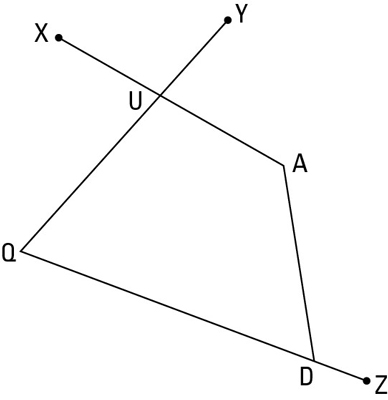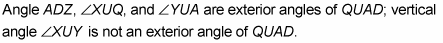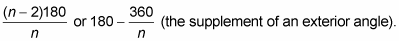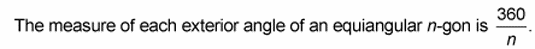##### Geometry For DummiesEverything you need to know about a polygon doesn’t necessarily fall within its sides. You may need to find exterior angles as well as interior angles when working with polygons:• Interior angle: An interior angle of a polygon is an angle inside the polygon at one of its vertices. Angle Q is an interior angle of quadrilateral QUAD.

• Exterior angle: An exterior angle of a polygon is an angle outside the polygon formed by one of its sides and the extension of an adjacent side.Interior and exterior angle formulas:
• The sum of the measures of the interior angles of a polygon with n sides is (n – 2)180.

• The measure of each interior angle of an equiangular n-gon is• If you count one exterior angle at each vertex, the sum of the measures of the exterior angles of a polygon is always 360°.Check here for more practice.